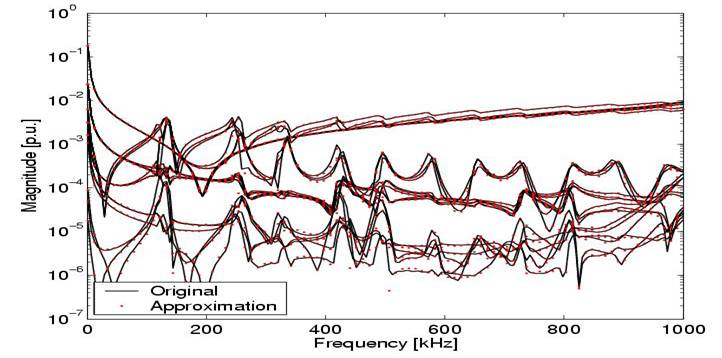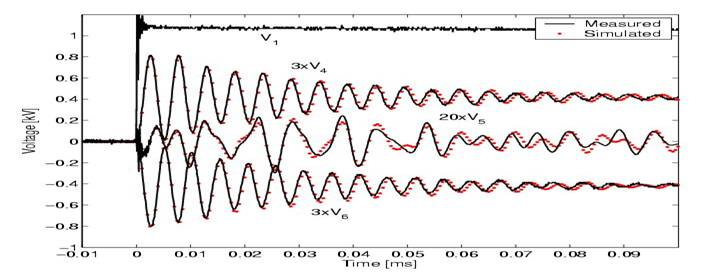Norsk English

# Vector Fitting

## Example

The 6x6 terminal admittance matrix Y of a rectifier transformer was measured in the frequency domain using a network analyzer. A state space model (60 poles per column) was calculated using Vector Fitting (vfit.zip), see [3.5].

The 6x6 terminal admittance matrix Y of a rectifier transformer was measured in the frequency domain using a network analyzer. A state space model (60 poles per column) was calculated using Vector Fitting (vfit.zip), see [3.5].Terminal voltages were calculated in the time domain using recursive convolutions and compared to measured waveforms.